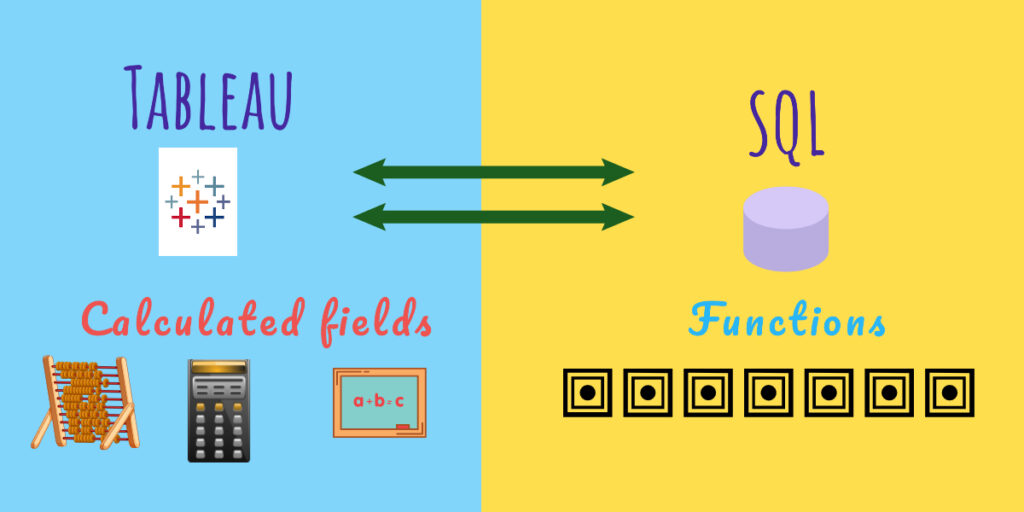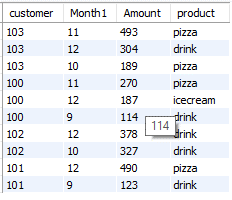# SQL Equivalent Tableau calculated fields## Introduction

Structured Query Language is also known as SQL is one of the most commonly used languages for storing and retrieving data from a Relational Data Base. They are also used for managing the Data present inside the database management system.

Tableau is the fastest growing business intelligence tool in the world which is used for processing, analyzing, and deriving insights from the data which is available from various data sources.

There are several types of calculated expressions in Tableau like Table Calculations, Basic Calculations, and LOD(Level Of Detail Expressions).

Hence in this article, we are going to see how to convert a few basic aggregated and string functions SQL expressions to Tableau calculated field expressions.

### Specifications

• Data: Retailer Dataset
• Environment: MySql and Tableau## Aggregate Functions:

Aggregate functions are the ones that operate on all rows of data that are present in the specified columns. These columns are then combined and clubbed to return a single value based on the summary indicator which has been provided.

Now let’s dive deep into a few basic aggregate functions.

### Sum

This is the most commonly used aggregate function wherein the values present in various rows are added together. It returns a single value as the output based on the values passed in a column. The output of the SQL query and its Tableau equivalent is shown below

### Average

The average function computes the arithmetic mean of the array of values that are passed through it.The output of the SQL query and its Tableau expression is represented below

### Minimum

The Min function compares all the values and returns the minimum value among the set of values in the given array. It works on strings as well by returning the minimum value based on the sorting sequence. The output of the SQL query and its Tableau expression is given below.

### Maximum

The Max function compares all the values and returns the maximum value among the set of values in the given array. It works on strings as well by returning the maximum value based on the sorting sequence. The output of the SQL query and its Tableau expression is given below

### Count

The Count function aggregates the sum of the count of occurrences present in each value of the given array. It works on both the continuous as well as categorical data types. The output of the SQL query and its Tableau expression is given below.

### Count Distinct

The Count Distinct function aggregates the sum of the distinct count of occurrences of the values present in the given array. It works on both the continuous as well as categorical data types. The output of the SQL query and its Tableau expression is given below.

### Group By

The group by is one of the paramount and most commonly used aggregate functions. It is used to combine one or more columns and later used in combination with the about mentioned functions to get values based on individual groupings of values. The output of the SQL query and its Tableau expression is given below

## Non Aggregate Functions

Non Aggregate functions work on each record independently rather than operating on the whole column cumulatively.

Many kinds of non-aggregate functions are used in data manipulation and data cleaning. We are going to take a look at few basic and Cardinal string functions which are commonly used to wrangle and analyze the data.

Numbers are always fun to work with now let’s see a few basic numeric functions.

## Number Functions

Number functions or numerical functions work on fields containing continuous variables. Although there are many numeric functions let us take a look at the most common ones.

### Absolute Function

The absolute function returns the absolute value of the continuous variable mentioned within the function. The output of the SQL query and its Tableau expression is given below.

For example, Absolute(-7)=7 and Absolute(80)=80.

### Ceiling Function

The Ceil function as it is known is one of the most commonly used numeric functions in tableau and is analogous to the round-off function. The ceil function returns the value greater or equal to the value of the next integer passed within the function. Shown below is an example of this along with the SQL query and Tableau expression.

For example: Ceil(3.14)=4

### Floor Function

The Floor function is another widely used numeric function and it is similar to the ceil function. The ceil function returns the value lesser or equal to the value of the previous integer passed within the function. Below is an example along with the SQL Query and its Tableau Equivalent.

Example: Floor(3.14)=3

### Square Root Function

The square root function returns the square root of the value which is passed as the argument within the function. The SQL query and Tableau equivalent is shown below.

Example: SquareRoot(2)=1.414

### Square Function

The square function returns the arithmetic squared value of the column/argument passed within the function. The SQL query and its Tableau equivalent are given below.

For Example Square(12)=144

Now let us move into the final and most interesting part of this blog the string functions.

## String Functions

String functions work on characters, words, or groups of words. There are 6 main string or literal functions namely concatenate, length, substr, position and replace. Despite numerous other functions being present, these are the basic and foremost important functions that are more predominantly used in creating derived values.

### Length Function

The length function returns the number of characters present in a string. It might be a character a word or a group of words.

Example: Length(red)=3

### Substr Function

This function returns the part of a string from the given start point to the endpoint. The MID function in Tableau performs the same function as substr in SQL.

### Concatenate

This function concatenates the two input columns. The Concat function is used in SQL and the + operator is used in Tableau.

That’s all about the basic aggregate and non-aggregate SQL equivalent functions in Tableau.

We’ll be taking a closer look at the other functions in my upcoming articles.

## Author

•### 1 thought on “SQL Equivalent Tableau calculated fields”

1.Very Good Article Dinesh